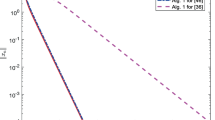# An approach to characterizing $$\epsilon$$-solution sets of convex programs

## Abstract

In this paper, we propose an approach to characterizing $${\epsilon}$$-solution sets of convex programs with a given $${\epsilon} >0$$. The results are divided into two parts. The first one is devoted to establishing the expressions of $${\epsilon}$$-solution sets of a class of convex infinite programs. The representation is given based on the study of relationships among the following three sets: the set of Lagrange multipliers corresponding to a given $${\epsilon}$$-solution, the set of $${\epsilon}$$-solutions of the dual problem corresponding, and the set of $${\epsilon}$$-Kuhn–Tucker vectors associated with the problem in consideration. The second one is devoted to some special cases: the $${\epsilon}$$-solution sets of convex programs that have set constraints and the almost $${\epsilon}$$-solution sets of convex programs that have finite convex constraints. Several examples are given.

This is a preview of subscription content, log in via an institution to check access.

Price excludes VAT (USA)
Tax calculation will be finalised during checkout.

### Similar content being viewed by others### Using Double Inertial Steps Into the Single Projection Method with Non-monotonic Step Sizes for Solving Pseudomontone Variational Inequalities

Duong Viet Thong, Xiao-Huan Li, … Hoang Thi Thanh Tam

Livinus U. Uko

### On the Existence of Solutions for Weak Nonlinear Bilevel Optimization Problems

Houda Keraoui, Fatima-Ezzahra Saissi & Abdelmalek Aboussoror

## References

• Aubin J-P, Vinter RB (2007) Convex Analysis and Optimization. Pitman Advanced Publishing Program, Boston

• Burachik RS, Jeyakumar V (2005) A new geometric condition for Fenchel’s duality in infinite dimensional spaces. Math Program 104:229–233

• Burke JV, Ferris M (1991) Characterization of solution sets of convex programs. Oper Res Lett 10:57–60

• Dinh N, Son TQ (2007) Approximate optimality conditions and duality for convex infinite programming problems. J. Sciences & Technology Development 10:29–38

• Dinh N, Goberna MA, López MA, Son TQ (2007) New Farkas-type constraint qualifications in convex infinite programming. ESAIM Control Optim Calc Var 13:580–597

• Draha A, Dutta J (2012) Optimality Conditions in Convex Optimization: A Finite Dimensional View. CRC Press Taylor Francis Group, New York

• Ivanov VI (2018) Characterizations of solution sets of differentiable quasiconvex programming problems. J Optim Theory Appl 181:144–162

• Jeyakumar V, Yang XQ (1995) Characterizing the solution sets of pseudo-linear programs. J Optim Theory Appl 87:747–755

• Jeyakumar V, Lee GM, Dinh N (2004) Lagrange multiplier conditions characterizing optimal solution sets of cone-constrained convex programs. J Optim Theory Appl 123:83–103

• Kim DS, Son TQ (2011) Characterizations of solution sets of a class of nonconvex semi-infinite programming problems. J Nonlinear Convex Anal 12:429–440

• Kim DS, Son TQ (2018) An approach to $${\epsilon}$$-duality theorems for nonconvex semi-infinite multiobjective optimization problems. Taiwanese J Math 22:1261–1287

• Lalitha CS, Mehta M (2009) Characterizations of solution sets of mathematical programs in terms of Lagrange multipliers. Optimization 58:995–1007

• Mangasarian OL (1988) A simple characterization of solution sets of convex programs. Oper Res Lett 7:21–26

• Scovel C, Hush D, Steinwart I (2007) Approximate duality. J Optim Theory Appl 135:429–443

• Sisarat N, Wangkeeree R, Lee GM (2020) Some characterizations of robust solution set for uncertain convex optimization problems with locally Lipschitz inequality constraints. J Ind Manag Optim 16:469–493

• Son TQ (2013) Refinements of $${\epsilon}$$-duality theorems for a nonconvex problem with in infinite number of constraints. J Nonlinear Anal Optim 4:61–70

• Son TQ, Dinh N (2008) Characterizations of optimal solution sets of convex infinite programs. TOP 16:147–163

• Son TQ, Kim DS (2013) $${\epsilon}$$-mixed type duality for nonconvex multiobjective programs with an infinite number of constraints. J Glob Optim 57:447–465

• Son TQ, Kim DS (2014) A new approach to characterize the solution set of a pseudoconvex programming problem. J Comput Appl Math 261:333–340

• Son TQ, Strodiot JJ, Nguyen VH (2009) $${\epsilon}$$-optimality and $${\epsilon}$$-Lagrangian duality for a nonconvex programming problem with an infinite number of constraints. J Optim Theory Appl 141:389–409

• Son TQ, Tuyen NV, Wen C-F (2020) Optimality conditions for approximate Pareto solutions of a nonsmooth vector optimization problem with an infinite number of constraints. Acta Math Vietnam 45:435–448

• Strodiot JJ, Nguyen VH, Heukems N (1983) $${\epsilon}$$-optimal solutions in nondifferentiable convex programming and some related questions. Math Program 25:307–328

• Tuyen NV (2021) Approximate solutions of interval-valued optimization problems. Invest Oper 42:223–237

• Tuyen NV, Xiao Y-B, Son TQ (2020) On approximate KKT optimality conditions for cone-constrained vector optimization problems. J Nonlinear Convex Anal 21:105–117

## Acknowledgements

The authors would like to thank the two anonymous reviewers, whose suggestions and comments improved the paper. The research of the first author was funded by Vietnam Ministry of Education and Training under Grant number B2021-SP2-06. A part of this work was done while he was visiting Vietnam Institute for Advanced Study in Mathematics (VIASM). He would like to thank VIASM for support and hospitality. The research of the second author was supported partially by the Grant MOST 108-2115-M-037-001, and the grant from Research Center for Nonlinear Analysis and Optimization Kaohsiung Medical University, Taiwan. The research of the third author was supported partially by Saigon University. He would also like to express his sincere thanks to the Department of Applied Mathematics, National Sun Yat-sen University, Kaohsiung, Taiwan, where parts of his research were carried out.

## Author information

Authors

### Corresponding author

Correspondence to T. Q. Son.